# Numbers - math word problems

#### Number of problems found: 1462

• DivisionWhich number in division 16 give 12 and the rest 3?
• Hazard game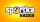In the Sportka hazard game, 6 numbers out of 49 are drawn. What is the probability that we will win: a) second prize (we guess 5 numbers correctly) b) the third prize (we guess 4 numbers correctly)?
• What areWhat are the four terms between 4 and 19?
• Two numbersFind the four times smaller number for numbers 12 and 36 and then add them.
• Find the 11Find the quotient of 229.12 and 12.32
• Goniometric form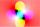Determine goniometric form of a complex number ?.
• Equation 11Solve equation: ?
• Find the meanFind the number between 13 and 29.
• Z7-I-4 stars 4949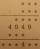Write instead of stars digits so the next write of product of the two numbers to be valid: ∗ ∗ ∗ · ∗ ∗ ∗ ∗ ∗ ∗ ∗ 4 9 4 9 ∗ ∗ ∗ ∗ ∗ ∗ 4 ∗ ∗
• Number train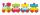The numbers 1,2,3,4,5,6,7,8 and 9 traveled by train. The train had three cars and each was carrying just three numbers. No. 1 rode in the first carriage, and in the last carriage was all odd numbers. The conductor calculated sum of the numbers in the firs
• Expression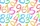If A=2 B=3 evaluate expression A(B+A) and multiply it by A
• Root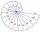Use law of square roots roots: ?
• Ratio v2Decrease in the ratio 12:16 number 13.2.
• Numbers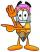By how many is the difference of numbers 8 and 34 less than its product?
• CotangentIf the angle α is acute, and cotg α = 1/3. Determine the value of sin α, cos α, tg α.
• Numbers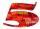Write smallest three-digit number, which in division 5 and 7 gives the rest 2.
• Divisible by fourAre 240 divisible by 4?
• Two numbersFind two numbers whose difference and ratio is 2.
• Octahedron - sumOn each wall of a regular octahedron is written one of the numbers 1, 2, 3, 4, 5, 6, 7 and 8, wherein on different sides are different numbers. For each wall John make the sum of the numbers written of three adjacent walls. Thus got eight sums, which also
• Cube root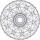Find cube root of 18

Do you have an interesting mathematical word problem that you can't solve it? Submit a math problem, and we can try to solve it.

We will send a solution to your e-mail address. Solved examples are also published here. Please enter the e-mail correctly and check whether you don't have a full mailbox.

Please do not submit problems from current active competitions such as Mathematical Olympiad, correspondence seminars etc...